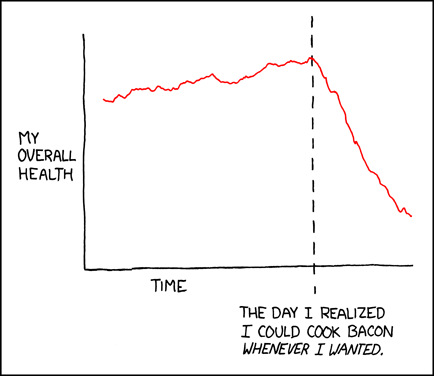# Tag Archives: Spiral

## Fibonacci…

I was recently approached to see if I could tinker about and create a short video associated with the Fibonacci series and more specifically the golden ratio for a maths MOOC that’s about to launch.  As per usual my inability to say no came into play and then I suddenly realised that I’ve never created a teaching video before and really what do I know about the Fibonacci series apart from fluffing about with a few Mathematica notebooks, and so blind panic set in… and with blind panic comes a quick trip to XKCD to check out the latest strips for a bit of inspiration…Now the golden ratio (φ) doesn’t sound like the sort of everyday mathematics that you would use will pushing a trolley around TESCO’s but the key thing you have to appreciate is that it’s a very sneaky number.  Things are that are proportioned using the golden ratio are naturally and inexplicably appealing to the eye, we find the ratio a thing of beauty even though most people don’t even know what it is.  It turns out that it’s used in everything from composing pleasing photographs (using the rule of thirds as an approximation) through to designing the Parthenon in Greece which is proportioned using this ratio.  It’s cool stuff, and to be frank if Mother Nature sees fit to use the principle to establish geometries in plants and other animals… who am I to argue?!

One of the things I like about XKCD are the graphs that they create, they have a certain charm about them and I love the style… and as sketchy as it looks, I’ve always struggled to replicate these diagrams using Visio or Omnigraffle.One of my other digital haunts is the Stack Exchange forum, which as a (very) amateur coder I constantly find myself sitting reading through in a sense of amazement at some of the nifty tricks that people pull to make some quite elegant and funky code.  One of the sub-forums I like there is the Mathematica one, which recently asked the question if it was possible to create XKCD graphs using Mathematica, a copy of the thread is here.  I’ve replicated the code below, as much to save it for myself should the thread ever get closed.

The author of this code has created some sample images using this code and I think they’re brilliant.But coming back to the reason I needed to create some diagrams, the fibonacci sequence and the golden ratio… starting with the golden ratio this is defined by the equation below.

$\varphi = \frac{{1 + \sqrt 5 }}{2}$

$\varphi = 1.61803…$

The reason that this number is expressed as a fraction is that it is an irrational number, which means it’s similar to π and the decimal places keep on repeating forever with no repetition and so it can’t accurately be approximated a decimal.  So how would you approximate this number? Well to start with you need to understand the Fibonacci sequence, which sounds quite grand and complex, but is simple once you understand the trick to determine the sequence… essentially you add together the previous two numbers in the sequence and this gives you the next number.  So to start with 1+0=1;  1+1=2; 2+1=3; 3+2=5; etc etc.

${\text{1, 1, 2, 3, 5, 8, 13, 21, 34, 55, 89, 144…}}$

But how does this get you to the golden ratio?  Well, if you divide one number, by the number before it you get a series of numbers that after a few iterations start to get close to the golden ratio (φ).

$1,2,\frac{3}{2},\frac{5}{3},\frac{8}{5},\frac{{13}}{8},\frac{{21}}{{13}},\frac{{34}}{{21}},\frac{{55}}{{34}},\frac{{89}}{{55}},\frac{{144}}{{89}}…$

Which shows that the number starts to approximate towards the golden ratio with a reasonable level of accuracy after about the fifth iteration or so (well reasonable to a simple engineering brain anyway).. but let’s not be blinded by numbers, let’s draw an XKCD style graph using our new mathematica code…

The last ImageSize term is important to get a reasonable resolution on the image when you export it from Mathematica for embedding into other documents, but the code above gives the graph below… which I think is quite a good attempt at a XKCD style diagram.Now that we can determine the Fibonacci sequence and the golden mean easily in Mathematica, we could start to see how these relate to the large sunflower seed heads that mother nature creates time after time…Just a single line of code will help us generate some nice pretty Golden Ratio inspired spirals in Mathematica which look strangely familiar.So it turns out that I did know enough about the Fibonacci sequence and the Golden Ratio to be almost dangerous… the trick’s going to be can I record a short YouTube video for a maths MOOC as I promised to a half decent standard?  We’ll have to wait and see… but now I know I can muck about with formatting diagrams and the code that goes along with the Golden Ratio in Mathematica I can start to mess around with tessalating spheres to create geodesic domes…And here’s a Mathematica graphic after following the procedure set out in this blog post.But I don’t want to go off another tangent just yet with Bucky Fuller… but he was inspired by nature and the cleanness of the geometry that the golden ratio presented.